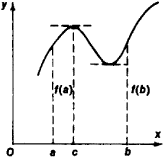Rolle's Theorem

Also found in: Dictionary, Wikipedia.

Rolle's theorem

[′rȯlz ‚thir·əm]
(mathematics)
If a function ƒ(x) is continuous on the closed interval [a, b ] and differentiable on the open interval (a, b) and if ƒ(a) = ƒ(b), then there exists x0, a <>x0<>b, such that ƒ′(x 0)=0.

Rolle’s Theorem

a theorem of mathematical analysis first stated by M. Rolle in 1690. According to this theorem, if the function f(x) is continuous on the closed interval [a, b], has a definite derivative within the interval, and takes on the equal values f(a) = f(b) at the ends of the interval, then the function’sFigure 1

derivative f’(x) vanishes at least once in the interval (a, b)—that is, there exists a c, a < c < b, such that f’(c) = 0. A corollary of the theorem provides that the derivative of a function has at least one zero between two successive zeros of the function. Geometrically, Rolle’s theorem is self-evident (see Figure 1). (See alsoDIFFERENTIAL CALCULUS.)

References in periodicals archive ?
Here is a way in which a person could come to believe Rolle's Theorem. Of course, it does not constitute a way of proving it.(6) But there may be discovery without proof.
(a) The following preliminary objection will occur to anyone who is familiar with the standard proof of Rolle's Theorem.(7) From the supposition that the curve rises smoothly and falls again over [a,b] it was inferred that the gradient of the tangent to the curve must change gradually from positive to negative at least once, as would happen if a segment of the curve had the shape of a path over a smooth hilltop.
A curve meeting the description of the previous paragraph would be uninterrupted and smooth, and so does not obviously violate the conditions of Rolle's Theorem.
If we reach Rolle's Theorem in the manner described above, the following is crucial to our becoming convinced that the derivative is at some point zero: when we visualize the line segment representing the tangent roll over the curve like a straight rigid rod, we visualize it assuming a horizontal position.
Rolle's Theorem concerns only those functions which have a derivative at every interior point; so the curve of the function must be smooth, unlike the function just described.
For these reasons the way of reaching Rolle's Theorem described in the previous section should not be regarded as an epistemically acceptable way of achieving belief in the theorem; it is not a way of making a genuine discovery.
This is much simpler than Rolle's Theorem; it does not involve the concept of the derivative of a function, and there is a fairly straightforward way of visualizing the situation.
Then we use the fact that h satisfies the conditions for Rolle's theorem to deduce that there is a point c in (a; b) such that h'(c) = 0, and the Mean Value Theorem follows.
(2) Describe how we can arrive at the function to which Rolle's Theorem is applied to yield the Cauchy Mean Value Theorem holds for P(t) on AC when t [member of] [[pi]/2, [pi]].
We sketch the graph of [x(t), y(t)] and line segment AC below in Figure 3 and we write the equation of the thick curve Q(t) as [x(t), [y.sub.1](t)], which is what we would like to find and where we will apply Rolle's Theorem later.
Site: Follow: Share:
Open / Close